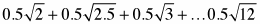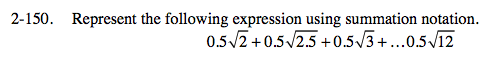### Home > PC > Chapter 2 > Lesson 2.3.6 > Problem2-150

2-150.
1. Represent the following expression using summation notation. Homework Help ✎$\displaystyle\sum\limits_{k=0}^{20}0.5\sqrt{0.5\textit{k}+2}$

Since all of the terms have a 0.5 multiplier, factor that out.

How many terms are there?

Decide if you will start at 0 or start at 1.

$0.5\displaystyle\sum$

21

$\displaystyle\sum\limits_{k=1}^{21}0.5\sqrt{0.5\textit{k}+1.5}$